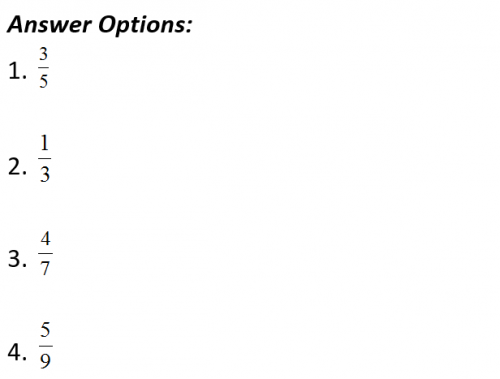### Wordpandit Question of the day

Question: There are three pipes, A, B and C, opening into a tank. Each pipe can be used to fill or empty the tank at the same respective rate. The ratio of the rates of the three pipes at which they either fill or empty the tank is 2: 3: 4. Pipes A and B, working together, take 3 hours to fill the tank when both are used as inlet pipes. In the first, second and third hours of operation, the pipes, A, B and C respectively are used as emptying pipes, while the other two pipes are used as filling pipes. What is the fraction of the tank filled at the end of the three hours?#### Solution posted below. Click on the plus sign to view the solution.

Since the rate of filling of pipes is in the ratio 2: 3: 4, let us assume that pipe A fills 2 lit/hr, pipe B fills 3 lit/hr and pipe C fills 4 lit/hr.

Now pipes A and B fill 5 lit/hr, when both are open, so in 3 hours pipes A and B will fill 15 liter.

Let the capacity of the tank be 15 liter

So, the water filled in first hour = 3 + 4 – 2 = 5 liter.

In the second hour, the water filled = 4 + 2 – 3 = 3 liter

In the third hour, the water filled will be = 2 + 3 – 4 = 1 litre

So in 3 hours, total water filled = 5 + 3 + 1 = 9 liter.

So, the fraction of tank that is filled = 9/15 =3/5